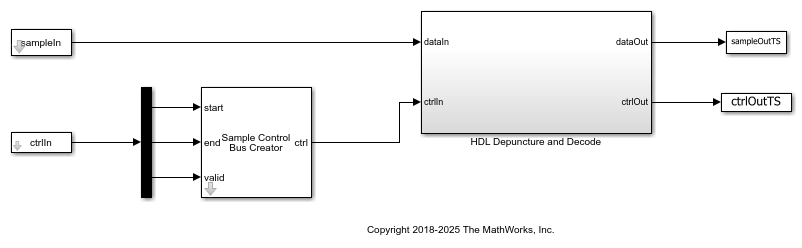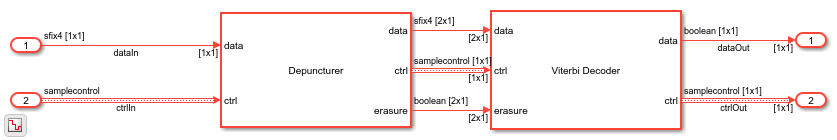# Depuncture and Decode Streaming Samples

This example shows how to use the hardware-friendly Depuncturer block and Viterbi Decoder block to decode samples encoded at WLAN code rates.

Generate input samples in MATLAB® by encoding random data, BPSK-modulating the samples, applying a channel model, demodulating the samples, and creating received soft-decision bits. Then, import the soft-decision bits into a Simulink® model to depuncture and decode the samples. Export the result of the Simulink simulation back to MATLAB and compare it against the original input samples.

The example model supports HDL code generation for the HDL Depuncture and Decode subsystem.

```modelname = 'ltehdlViterbiDecoderModel'; open_system(modelname); ```### Set Up Code Rate Parameters

Set up workspace variables that describe the code rate. The Viterbi Decoder block supports constraint lengths in the range [3,9] and polynomial lengths in the range [2,7].

Choose a traceback depth in the range [3,128]. For non-punctured samples, the recommended depth is 5 times the constraintLength. For punctured samples, the recommended depth is 10 times the constraintLength.

Starting from a code rate of 1/2, IEEE 802.11 WLAN specifies three puncturing patterns to generate three additional code rates. Choose one of these code rates, and then set the frame size and puncturing pattern based on that rate. You can also choose the unpunctured code rate of 1/2.

IEEE 802.11 WLAN specifies different modulation types for different code rates and uses `'Terminated'` mode. This example uses BPSK modulation for all rates and can run with `'Terminated'` or `'Truncated'` operation mode. The blocks also support `'Continuous'` mode, but it is not included in this example.

```constraintLength = 7; codeGenerator = [133 171]; opMode = 'Terminated'; tracebackDepth = 10*constraintLength; trellis = poly2trellis(constraintLength,... codeGenerator); % IEEE 802.11n-2009 WLAN 1/2 (7, [133 171]) % Rate Puncture Pattern Maximum Frame Size % 1/2 [1;1;1;1] 2592 % 2/3 [1;1;1;0] 1728 % 3/4 [1;1;1;0;0;1] 1944 % 5/6 [1;1;1;0;0;1;1;0;0;1] 2160 codeRate = 3/4; if (codeRate == 2/3) puncVector = logical([1;1;1;0]); frameSize = 1728; elseif (codeRate == 3/4) puncVector = logical([1;1;1;0;0;1]); frameSize = 1944; elseif (codeRate == 5/6) puncVector = logical([1;1;1;0;0;1;1;0;0;1]); frameSize = 2160; else % codeRate == 1/2 puncVector = logical([1;1;1;1]); frameSize = 2592; end if strcmpi(opMode,'Terminated') % Terminate the state at the end of the frame tailLen = constraintLength-1; else % Truncated mode tailLen = 0; end ```

### Generate Samples for Decoding

Use Communications Toolbox™ functions and System objects to generate encoded samples and apply channel noise. Demodulate the received samples, and create soft-decision values for each sample.

```EbNo = 10; EcNo = EbNo - 10*log10(numel(codeGenerator)); numFrames = 5; numSoftBits = 4; txMessages = cell(1,numFrames); rxSoftMessages = cell(1,numFrames); No = 10^((-EcNo)/10); quantStepSize = sqrt(No/2^numSoftBits); modulator = comm.BPSKModulator; channel = comm.AWGNChannel('EbNo',EcNo); demodulator = comm.BPSKDemodulator('DecisionMethod','Log-likelihood ratio'); for ii = 1:numFrames txMessages{ii} = [randn(frameSize - tailLen,1) zeros(tailLen,1)]>0; % Convolutional encoding and puncturing txCodeword = convenc(txMessages{ii},trellis,puncVector); % Modulation modOut = modulator.step(txCodeword); % Channel chanOut = channel.step(modOut); % Demodulation demodOut = -demodulator.step(chanOut)/4; % Convert to soft-decision values rxSoftMessagesDouble = demodOut./quantStepSize; rxSoftMessages{ii} = fi(rxSoftMessagesDouble,1,numSoftBits,0); end ```

### Set Up Variables for Simulink Simulation

The Simulink model requires streaming samples with accompanying control signals. Use the `whdlFramesToSamples` function to convert the framed `rxSoftMessages` to streaming samples and generate the matching control signals.

Calculate the required simulation time from the latency of the depuncture and decoder blocks.

```samplesizeIn = 1; idlecyclesbetweensamples = 0; idlecyclesbetweenframes = 0; if strcmpi(opMode,'Truncated') % Truncated mode requires a gap between frames of at least constraintLength-1 idlecyclesbetweenframes = constraintLength - 1; end [sampleIn,ctrlIn] = whdlFramesToSamples(rxSoftMessages, ... idlecyclesbetweensamples,idlecyclesbetweenframes,samplesizeIn); depunLatency = 6; vitLatency = 4*tracebackDepth + constraintLength + 13; latency = vitLatency + depunLatency; simTime = size(ctrlIn,1) + latency; sampletime = 1; ```

Call the Simulink model to depuncture and decode the samples. The model exports the decoded samples to the MATLAB workspace. The Depuncture and Viterbi Decoder block parameters are configured using workspace variables. Because Operation mode is a list parameter, use `set_param` to assign the workspace value.

Convert the streaming samples back to framed data for comparison.

```set_param([modelname '/HDL Depuncture and Decode'],'Open','on'); set_param([modelname '/HDL Depuncture and Decode/Viterbi Decoder'],... 'TerminationMethod',opMode); sim(modelname); sampleOut = squeeze(sampleOutTS.Data); ctrlOut = [squeeze(ctrlOutTS.start.Data) ... squeeze(ctrlOutTS.end.Data) ... squeeze(ctrlOutTS.valid.Data)]; rxMessages = whdlSamplesToFrames(sampleOut,ctrlOut); ```
```Maximum frame size computed to be 1944 samples. ```### Verify Results

Compare the output samples against the generated input samples.

```fprintf('\nDecoded Samples\n'); for ii = 1:numFrames numBitsErr = sum(xor(txMessages{ii},rxMessages{ii})); fprintf('Frame #%d: %d bits mismatch \n',ii,numBitsErr); end ```
```Decoded Samples Frame #1: 0 bits mismatch Frame #2: 0 bits mismatch Frame #3: 0 bits mismatch Frame #4: 0 bits mismatch Frame #5: 0 bits mismatch ```